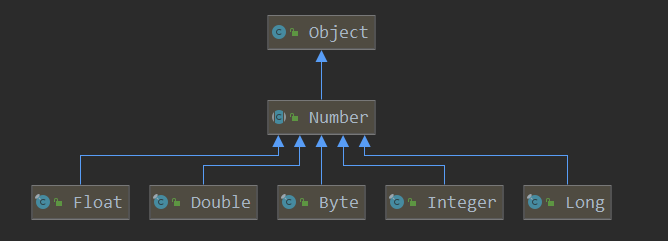# 二、泛型的意义

Java 在引入泛型之前，表示可变对象，通常使用 Object 来实现，但是在进行类型强制转换时存在安全风险。有了泛型后：

• 编译期间确定类型，保证类型安全，放的是什么，取的也是什么，不用担心抛出 ClassCastException 异常。

• 提升可读性，从编码阶段就显式地知道泛型集合、泛型方法等处理的对象类型是什么。

• 泛型合并了同类型的处理代码提高代码的重用率，增加程序的通用灵活性。

public static void method1() {
List list = new ArrayList();
​
for (Object o : list) {
System.out.println(o.getClass());
}
}

class java.lang.Integer
class java.lang.String
class java.lang.Object

public static void method2() {
List<String> list = new ArrayList();
​
for (String s : arrayList) {
System.out.println(s);
}
}

# 三、泛型的表示

• 普通符号 <T>

• 无边界通配符 <?>

• 上界通配符 <? extends E> 父类是 E

• 下界通配符 <? super E> 是 E 的父类

# 四、泛型的使用

## 4.1 泛型类

public class Test<T> {

private T data;
​
public T getData() {
return data;
}
​
/** 这种写法是错误的，提示 T 未定义 */
/*public static T get() {
return null;
}*/
/** 正确写法，该方法上的 T 和类上的 T 虽然一样，但是是两个指代，可以完全相同，互不影响 */
public static <T> T get() {
return null;
}

public void setData(T data) {
this.data = data;
}
}

## 4.2 泛型方法

public class Test {
​
public static <String, T, Hncboy> String get(String string, Hncboy hncboy) {
return string;
}
​
public static void main(String[] args) {
Integer first = 666;
Double second = 888.0;
Integer result = get(first, second);
System.out.println(result);
}
}

## 4.3 泛型通配符

? 为泛型非限定通配符，表示类型未知，不用声明，可以匹配任意的类。该通配符只能读，不能写，且不对返回值进行操作。也可以将非限定通配符出现的地方用普通泛型标识，不过使用通配符更简洁。举个例子：

test1() 是通过通配符来输出集合的每一个元素的，test2() 和 test1() 的作用一样，只不过将通配符用 <T> 来代替了；test3() 用来演示集合在通配符的情况下写操作，发现编译器报错，int 和 String 都不属于 ? 类型，当然放不进集合，因为所有类都有 null 元素，所以可以放进集合。比如主函数传的是 List<Double>，而想要在集合里添加一个 String，这是不可能的；test4() 的写法也是错的，? 是不确定，返回值返回不了；test5() 的用法使用来比较 List<Object> 和 List<?> 的，在主函数里调用 test5(list) 报错的，显示 java: 不兼容的类型: java.util.List<java.lang.Integer>无法转换为java.util.List<java.lang.Object>，因为 List<Integer> 不是 List<Object> 的子类。

public class Test {
​
public static void test1(List<?> list) {
for (int i = 0; i < list.size(); i++) {
System.out.println(list.get(i));
}
}
​
public static <T> void test2(List<T> list) {
for (int i = 0; i < list.size(); i++) {
System.out.println(list.get(i));
}
}
​
public static void test3(List<?> list) {
}
​
/*public static ? test4(List<?> list) {
return null;
}*/

public static void test5(List<Object> list) {
for (int i = 0; i < list.size(); i++) {
System.out.println(list.get(i));
}
}

public static void main(String[] args) {
List<Integer> list = new ArrayList<>();
test1(list);
test2(list);
//test5(list);
}
}<? extends Number> 表示类型为 Number 或 Number 的子类，<? super Integer> 表示类型为 Integer 或 Integer 的父类，举个例子，method1 方法测试是上边界 Number，由于 arrayList1 和 arrayList2 的泛型都为 Number 或其子类，所以可以插入成功，而 arrayList3 的类型 String 和 Number 无关，因此编译报错。method2 方法测试的是下边界 Integer，由于 arrayList4，arrayList5 和 arrayList7 种的类型 Integer、Object 和 Number 都为 Integer 的父类，所以插入成功，而 arrayList7 的类型 Double，因此插入失败。

public class Generic {

public static void main(String[] args) {
ArrayList<Integer> arrayList1 = new ArrayList<>();
ArrayList<Number> arrayList2 = new ArrayList<>();
ArrayList<String> arrayList3 = new ArrayList<>();
method1(arrayList1);
method1(arrayList2);
//method1(arrayList3);

ArrayList<Integer> arrayList4 = new ArrayList<>();
ArrayList<Object> arrayList5 = new ArrayList<>();
ArrayList<Number> arrayList6 = new ArrayList<>();
ArrayList<Double> arrayList7 = new ArrayList<>();
method2(arrayList4);
method2(arrayList5);
method2(arrayList6);
//method2(arrayList7)
}

public static void method1(ArrayList<? extends Number> arrayList) {
}

public static void method2(ArrayList<? super Integer> arrayList) {
}
}

## 4.4 泛型接口

public interface CalcGeneric<T> {
}
​
public class CalculatorGeneric<T> implements CalcGeneric<T> {
​
@Override
public T add(T num1, T num2) {
return null;
}
}

## 4.5 泛型数组

public class Test {
​
public static void main(String[] args) {
Number[] numbers = new Integer; // 1
// java.lang.ArrayStoreException: java.lang.Double
numbers = new Double(1); // 2
//List<String>[] list = new ArrayList<String>; // 3
List<String>[] list2 = new ArrayList; // 4
//List<Number> list3 = new ArrayList<Integer>(); // 5
}
}

## 4.6 泛型擦除

class java.util.ArrayList
class java.util.ArrayList
true
public static void method1() {
List<Integer> integerArrayList = new ArrayList();
List<String> stringArrayList = new ArrayList();
​
System.out.println(integerArrayList.getClass());
System.out.println(stringArrayList.getClass());
System.out.println(integerArrayList.getClass() == stringArrayList.getClass());
}

public static method1()V
L0
LINENUMBER 14 L0
NEW java/util/ArrayList
DUP
INVOKESPECIAL java/util/ArrayList.<init> ()V
ASTORE 0
L1
LINENUMBER 15 L1
NEW java/util/ArrayList
DUP
INVOKESPECIAL java/util/ArrayList.<init> ()V
ASTORE 1

public static void method4() throws Exception {
ArrayList<String> stringArrayList = new ArrayList<>();
System.out.println("之前长度：" + stringArrayList.size());
​
// 通过反射增加元素
Class<?> clazz = stringArrayList.getClass();
method.invoke(stringArrayList, 60);
​
System.out.println("之后长度：" + stringArrayList.size());
// 存的还是 Integer 类型
// java.lang.ClassCastException: java.lang.Integer cannot be cast to java.lang.String
for (int i = 0; i < stringArrayList.size(); i++) {
System.out.println(stringArrayList.get(i).getClass());
}
}

# 五、总结

• 数组不支持泛型

• 泛型的类型不能为基础数据类型

• 泛型只在编译阶段有效

Java 编程思想

java 泛型详解

posted on 2019-12-17 16:37  hncboy  阅读(781)  评论(0编辑  收藏  举报